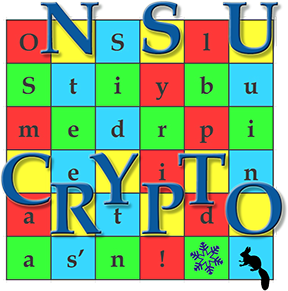### Additional information

• The Enigma machine used in the problem follows the pattern:
(Rot_i^{-1}. R^{-1}). (Rot_j^{-1}. M^{-1}).(Rot_k^{-1}. L^{-1}). Reflect. (L. Rot_k). (M. Rot_j). (R. Rot_i). (Plaintext) => Ciphertext
• Here "." denotes function composition; R, M, L stand for the right, middle and left rotors; Rot_i denotes the alphabet's rotation by i.
• Thus, once an output has been obtained from the first rotor, it is directly fed into the second rotor (after taking into consideration the fact that the second rotor has been rotated with "j" positions) and then into the third.